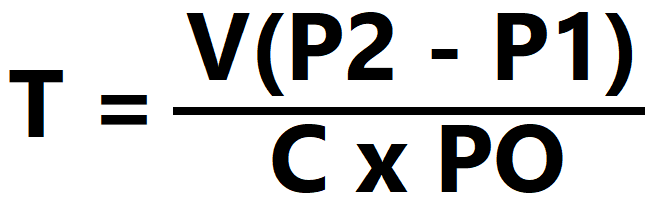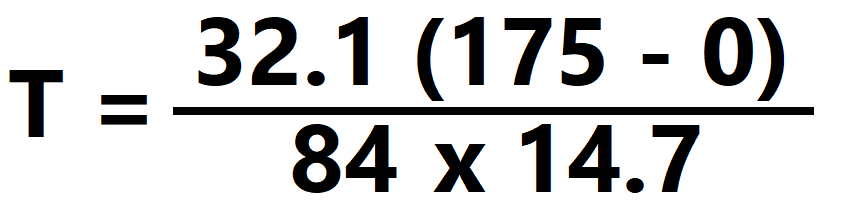T = Time (Min)

P2 = Final Receiver Pressure (PSI)

P2 = Initial Receiver Pressure (PSI)

C = Compressor Production (CFM)

PO = Atmospheric Pressure (PSI) – Typically 14.7

**** Volume (ft^3) = Gallons / 7.48

Example 1: How long will it take for a compressor with an output of 84 CFM to fill a 240 gallon tank at 175 PSI?

Start by converting gallons to ft^3: 240 / 7.48 = 32.1

Then complete the equation:## T = 4.55 min

Now that you know how the formula works, feel free to save time and use our calculator below